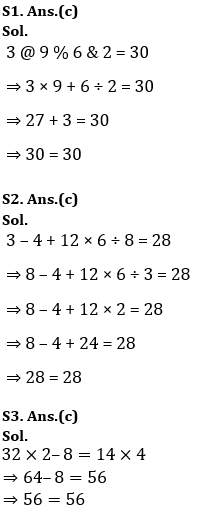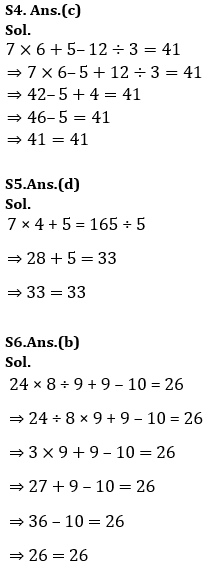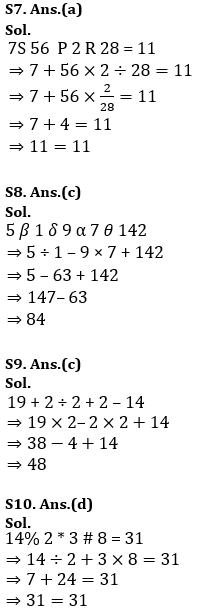Latest SSC jobs   »   Target SSC CGL | 10,000+ Questions...

# Target SSC CGL | 10,000+ Questions | Reasoning Questions For SSC CGL : Day 122

This is the new year with new goals, new experiences and lots of exams to be scheduled soon. SSC CGL has recently released the exam dates so now it is time to gear up your preparations and try hard to get success. ADDA247 never fails to deliver something new and fruitful for you all. This time also we are providing you the best study plan as well as a study material. We are here going to prepare your Reasoning section for the SSC CGL. In this article, we are providing you the details that how we are going to help you to clear the examination this year. We ADDA247 is going to provide you daily tests for all the subjects. The topic-wise quiz will be done from January till April. This will help you to get a deeper knowledge of all the topics and will prepare you thoroughly.

Q1.If “@” denotes “multiplied by”, “\$” denotes “subtracted from”, “%” denotes “added to” and “&” denotes “divided by”, then which of the following equation must be true?
(a) 7 % 9 & 3 \$ 5 = 4
(b) 4 % 3 @ 6 \$ 9 = 12
(c) 3 @ 9 % 6 & 2 = 30
(d) 6 % 9 @ 2 \$ 7 = 16

Q2.In the following question, correct the equation by interchanging two numbers.
3 – 4 + 12 × 6 ÷ 8 = 28
(a) 3 and 4
(b) 4 and 8
(c) 3 and 8
(d) 4 and 6

Q3.In the following question, by using which mathematical operators will the expression become correct?
32 ? 2 ? 8 ? 14 ? 4
(a) x, +, = and ÷
(b) +, +, = and x
(c) x, –, = and x
(d) +, x, = and x

Q4.In the following question, correct the equation by interchanging two signs.
7 × 6 + 5 – 12 ÷ 3 = 41
(a) + and ÷
(b) × and ÷
(c) + and –
(d) × and –

Q5.In the following question, by using which mathematical operators will the expression become correct?
7 ? 4 ? 5 ? 165 ? 5
(a) +, +, ÷ and =
(b) ×, ×, = and +
(c) ×, ÷, = and ÷
(d) ×, +, = and ÷

Q6.In the following question, correct the equation by interchanging two signs.
24 × 8 ÷ 9 + 9 – 10 = 26
(a) ÷ and –
(b) ÷ and ×
(c) × and –
(d) × and +

Q7.If “P” denotes “multiplied by”, “Q” denotes “subtracted from”, “S” denotes “added to” and “R” denotes “divided by”, then which of the following equation must be true?
(a) 7 S 56 P 2 R 28 = 11
(b) 36 R 6 P 2 S 4 = 19
(c) 64 R 8 P 3 S 6 = 72
(d) 36 R 9 S 4 P 2 = 14

Q8.If “θ” denotes “added to”, “δ” denotes “subtracted from”, “β” denotes “divided by” and “α” denotes “multiplied by”, then 5 β 1 δ 9 α 7 θ 142 = ?
(a) 114
(b) 98
(c) 84
(d) 125

Q9.If “÷” denotes “subtracted from”, “+” denotes “multiplied by”, “–” denotes “added to” and “×” denotes “divided by”, then19 + 2 ÷ 2 + 2 – 14 = ?
(a) 52
(b) 46
(c) 48
(d) 88

Q10.If “#” denotes “multiplied by”, “@” denotes “subtracted from”, “*” denotes “added to” and “%” denotes “divided by”, then which of the following equation must be true?
(a) 4 % 2 # 6 * 7 = 18
(b) 3 # 9 @ 4 # 6 = 4
(c) 5 # 6 % 3 @ 4 = 8
(d) 14 % 2 * 3 # 8 = 31

Solutions#### Congratulations!General Awareness & Science Capsule PDF